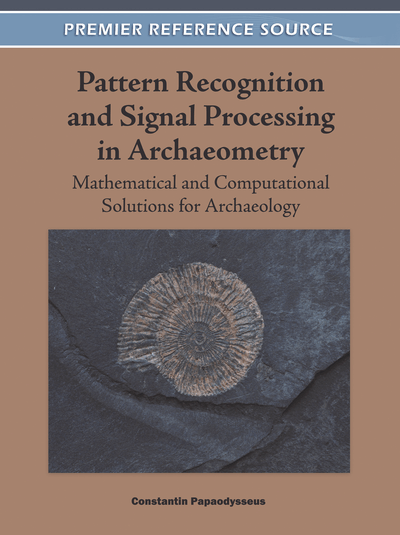# Applications of Computational and Model-Based Statistical Methodologies in Archaeology

Ioulia Papageorgiou (Athens University of Economics and Business, Greece)
Copyright: © 2012 |Pages: 26
DOI: 10.4018/978-1-60960-786-9.ch001

## Abstract

Quantitative Archaeology had a rapid development in the past few decades due to the parallel development of methodologies in Physics, Chemistry and Geology that can be implemented in archaeological findings and produce measurements on a number of variables. Those measurements form the data, the basis for a statistical analysis, which in turn can provide us with objective results and answers, within the prediction or estimation framework, about the archaeological findings. Exploratory statistical analysis was almost exclusively used initially for analyzing such data mainly because of their simplicity. The simplicity originates from the fact that exploratory techniques do not rely on any distribution assumption and conduct a non-parametric statistical analysis. However the recent development of the statistical methodology and the computing software allows us to make use of more sophisticated statistical techniques and obtain more informative results. We explore and present applications of three such techniques. The finite mixture approach for model based clustering, the latent class model and the Bayesian mixture of normal distributions with unknown number of components. All three methods can be used for identifying sub-groups in the sample and classify the items.
Chapter Preview
Top

## 1. Introduction

The need to draw conclusions on Archaeological findings lead scientists to seek for quantitative description of the data resulting to a large number of measurements, aiming to cover all different aspects of useful information the findings can provide. The set of measurements, called variables in statistical context, form the input of mathematical and statistical methodologies which in turn attempt to (i) exploit the data, (ii) combine the different part of information provided and (iii) subjectively derive conclusions that answer to questions archaeologists have posed.

The data set describing a problem in Archaeology usually has some particular properties that either point out specific categories of methods to be used or impose limitations in some others. For example the data set consisted of a number of measurements on some archaeological findings does not contain the dependent variable we are interested in. This variable can be for instance the date or the region that the artefact has been made, the person e.g. writer, ceramist, sculptor who has created it, etc. Therefore, modeling the variable under study (dependent variable) with respect to a number of covariates, independent variables, it is not an issue in this case. The dependent variable in problems from archaeology is a latent variable, a variable that we are not in a position to measure across the sampling units but is the underlying one that controls the variables we have measured. In general the typical data set in the archaeological context will be a set of variables that all are equivalent as all provide a small part of information and need to be treated simultaneously. The outcome of the analysis will be informative about the latent variable.

The statistical methods appropriate for such data sets are methods from multivariate statistical analysis and principal components analysis (PCA), cluster analysis (CA), factor analysis (FA), correspondence analysis (CORA), multidimensional scaling (MDS) and discriminant analysis (DA) are the most popular. Most of these methodologies are distribution-free methodologies or exploratory and use measures based on distances or degree of matching or geometrical representation to reveal the information that the data contain. A book of reference for statistical multivariate techniques with applications in Archaeology is Baxter (1994) and for the Bayesian approach is Buck et al (1996).

Another characteristic of the data sets in these applications is that the number of variables is usually larger than the number of items. The items are the findings from excavations and therefore its number is given and usually small. On the other hand we wish to keep all available variables assuming that all have at least a small part of contribution in information regarding the unknown variable. Alternatively, we need to carry out a subjective statistical selection of the most informative variables to the latent one(s) and keep this subset of variables for the analysis. As a consequence, the model-based methodologies that are based on an assumption about the distribution of the data have limited use in Archaeology, because the data will not be sufficient to estimate the large number of the unknown parameters in the problem caused of their high dimensionally. The parameters of the problem are the parameters of the assumed multivariate distribution and the situation gets even more complex when heterogeneity occurs in the data, something which is quite common in this context. For example, 2 different origins for ceramics or 3 different writers for inscriptions represent two or three respectively, different sub-populations that are described from distributions which differ in parameters. If m is the number of parameters to be estimated in a population and there are k sub-populations, m×k are the parameters in total that have to be estimated from the sample. For a realistic example, we can see that even assuming normal distribution for a data set with 2 groups and p=10 variables, the number of parameters will be. The first part corresponds to the mean vectors parameters and the second to the variance covariance matrices in their general form where neither sphericity nor other assumption is made.

Mainly these two particular characteristics of the data are the reason why exploratory techniques rather than model based have been used in Archaeology in the last few decades (Baxter, 2006).

## Complete Chapter List

Search this Book:
Reset